## TN State Board 12th Physics Important Questions Chapter 7 Dual Nature of Radiation and Matter

Question 1.
Do we the photons have same mass? If not why?
No, Mass of photon = $$\frac{\mathrm{E}}{m^{2}}=\frac{h v}{c^{2}}$$
Differrent radiations have different frequencies. So, their photons will have different mass.

Question 2.
Which photon is more energetic – a red one or a violet one?
Since frequency of violet is greater than that of red, i.e., υviolet > υred
Violet photon has more energy because energy of a photon E = hυ.Question 3.
What happens to the wavelength of a photon after it colloids with an electron?\
A photon transfers a part of in energy to the colloiding electron, so its energy decreases and consequently wavelength increases.

Question 4.
Out of microwaves, ultra-violet rays and infrared rays, which radiations will be most effective for emission of electrons from a metallic surface?
Ultra-violet rays are most effective for photo electric emission because they have highest frequency and hence most energetic.

Question 5.
On What principle is an electron microscope based?
An electron microscope exploits the wave nature of an accelerated beam of electrons to provide high magnifying and resolving powers.Question 6.
Can X-rays cause photo electric effect?
Yes, X-rays can cause photo electric effect in sodium, zinc and copper.

Question 7.
Work function of aluminium is 4.2 eV. If two photons each of energy 2.5 eV are incident on its surface, will the emission of electrons take place? Justify.
No, energy of a single photon must be greater than the work function of the metal for emission of a photo electron.

Question 8.
Define stopping potential.
The minimum negative (retarding) potential given to the anode for which the photo electric current becomes zero is called the cut-off or stopping potential.Question 9.
How X-rays are produced?
Whenever fast moving electrons are suddenly stopped by a metal target like copper. X-rays are produced.

Question 10.
Write down the applications of X-rays.
X-rays are being used in the following fields.
(i) Medical diagnosis:
X-rays can pass through flesh more easily than through bones. Thus an X-ray radiograph containing a deep shadow of the bones and a light shadow of the flesh may be obtained. X-ray radiographs are used to detect fractures, foreign bodies, diseased organs etc…

(ii) Medical therapy:
Since X-rays are used to kill diseased tissues, they are employed to cure skin diseases, malignant tumours etc.

(iii) Industry:
X-rays are used to check for flaws in welded joints, motor tyres, tennis balls and wood. At the custom post, they are used for detection of contraband goods.

(iv) Scientific research:
X-ray diffraction is important tool to study the structure of the crystalline materials and molecules in crystals.Question 11.
How X-rays are produced?
X-rays are produced in X-ray tube which is essentially a discharge tube as shown in figure.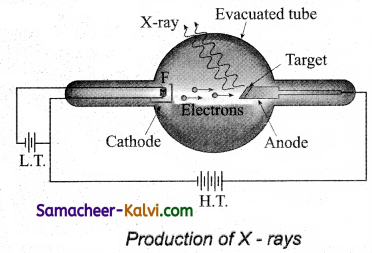A tungsten filament F is heated to incandescence by a battery. As a result, electrons are emitted from it by thermionic emission. The electrons are accelerated to high speeds by the voltage applied between the filament F and the anode. The target materials like tungsten, molybdenum are embedded in the face of the solid copper anode.

The face of the target is inclined at an angle with respect to the electron beam so that X-rays can leave the tube through its side. When high-speed electrons strike the target, they are decelerated suddenly and lose their kinetic energy. As a result, X-ray photons are produced. Since most of the kinetic energy of the bombarding electrons gets converted into heat, targets made of high melting point metals and a cooling system are usually employed.Question 12.
Explain the origin of continuous X-ray.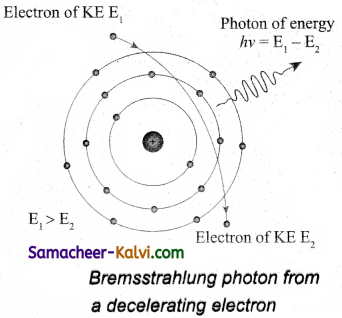When a fast moving electron penetrates and approaches a target nucleus, the interaction between the electron and the nucleus either accelerates or decelerates it which results in a change of path of the electron. The radiation produced from such decelerating electron is called braking radiation.

The energy of the photon emitted is equal to the loss of kinetic energy of the electron. Since an electron may lose part or all of its energy to the photon, the photons are emitted with all possible energies. The continuous X-ray spectrum is due to such radiations.

When an electron gives up all its energy, then the photon is emitted with highest frequency υ0 (or lowest wavelength λ0). The initial kinetic energy of an electron is given by eV where V is the accelerating voltage.
∴ eV = hυ0
eV = $$\frac{h c}{\lambda_{0}}$$

λ0 = $$\frac{h c}{\mathrm{eV}}$$

where λ0 is the cut-off wavelength, substituting the known values in the above equation, we get

λ0 = $$\frac{12400}{\mathrm{~V}}$$
The value of depends only on the accelerating potential and is same for all targets.Question 13.
Explain the origin of characteristic X-ray spectra.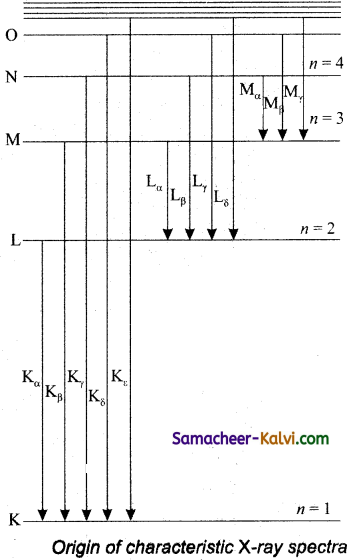X-ray spectra show some narrow peaks at some well-defined wavelengths when the target is hit by fast electrons. The line spectrum showing these peaks is Called characteristic X-ray spectrum. This is to electronic transitions within the atoms. When an energetic electron penetrates into the target atom and removes some of the K-shell electrons.

Then the electrons from outer orbits jump to fill up the vacancy so created in the K-shell. During the downward transition, the energy difference between the levels is given out in the form of X-ray photon of definite wavelength. Such wavelengths, characteristic of the target, constitute the line spectrum.

From the figure, it is evident that R-series of lines in the X-ray spectrum of an element arises due to the electronic transition from L, M, N… levels to the K- level. Similarly, the longer wavelength L-series originates when an L-electron is knocked out of the atom and the corresponding vacancy is filled by the electronic transition from M, N, O… and so on.

Question 14.
The threshold wavelength for sodium is 680nm, What is the photoelectric work function in eV.
(1 eV = 1.6 × 10-19 J, c = 3 × 108 ms-1)
Work function w = $$\frac{h v_{0}}{e}$$
= $$\frac{h c}{\lambda_{0} e}$$
= $$\frac{6.63 \times 10^{-34} \times 3 \times 10^{8}}{680 \times 10^{-9} \times 1.6 \times 10^{-19}}$$
= 1.825 eVQuestion 15.
Find the number of photons radiated per second when mean wavelength of light radiated by 100 W lamp is 5000 Å.
Power p = $$\frac{n h c}{\lambda t}$$
$$\frac{n}{t}=\frac{p_{\lambda}}{h c}$$
= $$\frac{100 \times 5000 \times 10^{-10}}{6.63 \times 10^{-34} \times 3 \times 10^{8}}$$

= 2.50 × 1020 photons

Question 16.
What is the frequency of a photo whose energy is 66.3eV.
E = 66.3 eV
= 66.3 × 1.6 × 10-19 J
Frequency υ = $$\frac{\mathrm{E}}{h}$$
= $$\frac{66.3 \times 1.6 \times 10^{-19}}{6.63 \times 10^{-34}}$$
= 1.6 × 1016 Hz

Question 17.
For a photosensitive surface, work function is 3.3 × 10-19 J. Find threshold frequency.
Threshold frequency υ0 = $$\frac{w_{0}}{h}$$
= $$\frac{3.5 \times 10^{-19}}{6.63 \times 10^{-34}}$$
= 5 × 1014 Hz.

Question 18.
The following table gives the value of work function for a few photo sensitive metals

 Metal Work function (eV) Na 1.92 K 2.15 M0 4.17

In each of these metal is exposed to radiations of wavelength 300 nm, which of them will not emit photoelectrons and why?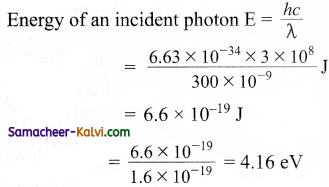M0 will not emit photoelectrons because the energy of incident photon is less than the work function of M0.Multiple Choice Questions:

Question 1.
As the intensity of incident light increases:
(a) photo electric current increases.
(b) photo electric current decreases.
(c) K.E of emitted photo electron decreases
(d) K.E of emitted photo electron increases
(a) photo electric current increases.

Question 2.
The stopping potential (V ) depends up on:
(a) intensity of incident light.
(b) angle of incidence.
(c) surface nature of the substance.
(d) does not depend upon intensity of incident light.
(d) does not depend upon intensity of incident light.

Question 3.
When wavelength of incident photon is decreased then:
(a) photo electric current increases.
(b) velocity of emitted photo electron increases.
(c) velocity of emitted photo electron decreases.
(d) velocity of photo electron does not change
(b) velocity of emitted photo electron increases.Question 4.
Of the following, the graph which represents the variation of energy (E) of a photon with wavelength (λ) of the radiation is:
(a)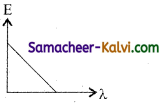(b)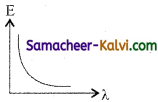(c)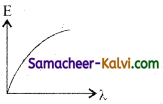(d)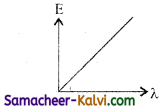(b)Question 5.
The figure shows the variation of photo current with anode potential for a photo sensitive surface for three different radiations. Let I , I, and I be the intensities and f, f, and f be the frequencies for the curve a, b and c respectively.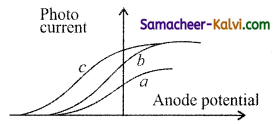(a) fa = fb and Ia ≠ Ib
(b) fa = fc and Ia = Ic
(c) fa = fb and Ia = Ib
(d) fa ≠ fb and Ia = Ib
(a) fa = fb and Ia ≠ IbQuestion 6.
According to Einstein’s photo electric equation, the graph between the kinetic energy of photo electrons ejected and the frequency of incident radiation is:
(a)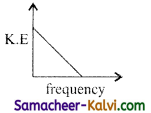(b)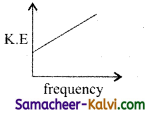(c)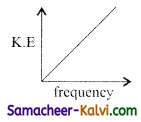(d)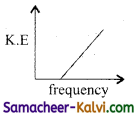(d)Question 7.
Light of two different frequencies whose photons have energies 1 eV and 2.5 eV respectively, successively illuminates a metal of work function 0.5 eV. The ratio of maximum K.E of the emitted electron will be:
(a) 1 : 5
(b) 1 : 4
(c) 1 : 1
(d) 1 : 2
(b) 1 : 4

Question 8.
Two identical photo-cathodes receive light of frequencies f1 and f2. If the velocities of the photo electrons of mass m coming out are respectively V1 and V2. Then:
(a) V1 – V2 = $$\left[\frac{2 h}{m}\left(f_{1}-f_{2}\right)\right]^{1 / 2}$$

(b) V12 – V22 = $$\frac{2 h}{m}\left(f_{1}-f_{2}\right)$$

(c) V1 + V2 = $$\left[\frac{2 h}{m}\left(f_{1}+f_{2}\right)\right]^{1 / 2}$$

(d) V12 + V22 = $$\frac{2 h}{m}\left(f_{1}+f_{2}\right)$$

(b) V12 – V22 = $$\frac{2 h}{m}\left(f_{1}-f_{2}\right)$$Question 9.
When radiation of wavelength λ is incident on a metallic surface, the stopping potential is 4.8 volts. If the same surface is illuminated with radiation of double the wavelength, then the stopping potential becomes 1.6 volts. Then the threshold wavelength for the surface is:
(a) 2λ
(b) 2λ
(c) 6λ
(d) 8λ
(b) 2λ

Question 10.
A photon and an electron have equal energy E. λphoton / λelectron is proportional to:
(a) $$\frac{1}{\mathrm{E}}$$
(b) √E
(c) $$\frac{1}{\sqrt{\mathrm{E}}}$$
(d) does not depend upon E
(c) $$\frac{1}{\sqrt{\mathrm{E}}}$$

Question 11.
If the work function of the metal is w and the frequency of incident light is v, there will be no emission of photoelectrons when:
(a) υ = $$\frac{w}{h}$$

(b) υ < $$\frac{w}{h}$$

(c) υ > $$\frac{w}{h}$$

(d) υ ≥ $$\frac{w}{h}$$
(c) υ > $$\frac{w}{h}$$Question 12.
If the distance of the source from a photo electric cell is varied, the saturation current I of the photo cell varies with the distance d as:
(a) I ∝ $$\frac{1}{d}$$
(b) I ∝ d2
(c) I ∝ $$\frac{1}{d^{2}}$$
(d) I ∝ d
(b) I ∝ d2

Question 13.
When light falls on a metal surface, the maximum kinetic energy of the emitted photo electrons depends upon:
(a) the time for which light falls on the metal
(b) frequency of the incident light
(c) intensity of the incident light
(d) velocity of the incident light
(b) frequency of the incident light

Question 14.
The figure shows different graphs between stopping potential (V0) and frequency (υ) for photosensitive surfaces of cesium, potassium, sodium and lithium. The plots are parallel. Correct ranking of the targets according to their work function greatest first will be:(a) (i) > (ii) > (iii) > (iv)
(b) (i) > (iii) > (ii) > (iv)
(c) (iv) > (iii) > (ii) > (i)
(d) (i) = (iii) > (ii) = (iv)
(c) (iv) > (iii) > (ii) > (I)Question 15.
What will be the ratio of de-Broglie wavelengths of proton and a-particle of same energy?
(a) 2 : 1
(b) 1 : 2
(c) 4 : 1
(d) 1 : 4
(a) 2 : 1

Question 16.
There are n1 protons of frequency v1 in a beam of light. In an equally energetic beam, there are n2 protons of frequency v2 Then the correct relation is:
(a) $$\frac{n_{1}}{n_{2}}$$ = 1

(b) $$\frac{n_{1}}{n_{2}}=\frac{\nu_{1}}{\nu_{2}}$$

(c) $$\frac{n_{1}}{n_{2}}=\frac{v_{2}}{v_{1}}$$

(d) $$\frac{n_{1}}{n_{2}}=\frac{v_{1}^{2}}{v_{2}^{2}}$$

(c) $$\frac{n_{1}}{n_{2}}=\frac{v_{2}}{v_{1}}$$

Question 17.
An electron and proton are moving with the same speed. Mass of proton is equal to 1836 times of mass of electron. The ratio of their de-Broglie wavelengths will be:
(a) 1
(b) 1836
(c) $$\frac{1}{1836}$$
(d) 918
(b) 1836Question 18.
The idea of wave associated with a moving electron was experimentally confirmed by:
(a) Davisson and Germer
(b) Hertz
(c) Dunnigton
(d) Rutherford
(a) Davisson and Germer

Question 19.
If the kinetic energy of the moving particle is E, then the de Broglie wavelength is:
(a) λ = $$\frac{h}{\sqrt{2 m \mathrm{E}}}$$

(b) λ = $$\frac{\sqrt{2 m \mathrm{E}}}{h}$$

(c) λ = $$h \sqrt{2 m \mathrm{E}}$$

(d) λ = $$\frac{h}{\mathrm{E} \sqrt{2 m}}$$

(a) λ = $$\frac{h}{\sqrt{2 m \mathrm{E}}}$$

Question 20.
The wave-particle duality was extended to particles as matter wave by:
(a) Max Planck
(b) Einstein
(c) Rutherford
(d) de Broglie
(d) de BroglieQuestion 21.
The particles emitted during photo electric effect are found to be:
(a) protons
(b) electrons
(c) neutrons
(d) positrons
(b) electrons

Question 22.
The important application of the de Broglie waves is:
(a) electron microscope
(b) compound microscope
(c) spectrometer
(d) CRO
(a) electron microscope

Question 23.
If m is the mass of a wave moving with velocity v then the wavelength of matter wave is given by λ =
(a) $$\frac{h}{m v^{2}}$$

(b) $$\frac{m v}{h}$$

(c) $$\frac{h}{m v}$$

(d) $$\frac{h v}{m}$$
(c) $$\frac{h}{m v}$$Question 24.
Which of the following statements is correct?
(a) The current in a photo cell increases with increasing frequency of light.
(b) The photo current is proportional to applied voltage.
(c) The photo current increases with increasing intensity of light.
(d) The stopping potential increases with increasing intensity of incident light.
(c) The photo current increases with increasing intensity of light.

Question 25.
Which of the following statement is not correct?
(a) photographic plates are sensitive to infrared rays.
(b) photographic plates are sensitive to ultraviolet rays.
(c) infrared rays are invisible but can cast shadows like visible light.
(d) infrared photons have more energy than photons of visible light.
(d) infrared photons have more energy than photons of visible light.

Question 26.
Photo electric effect can be explained by:
(a) corpuscular theory of light
(b) wave nature of light
(c) Bohr’s theory
(d) quantum theory of light
(d) quantum theory of lightQuestion 27.
Which of the following waves can produce photo electric effect?
(a) ultra sound
(b) infrared
(d) X – rays
(d) X – rays

Question 28.
If an electron and proton are propagating in the form of waves having the same X, it implies that they have the same:
(a) energy
(b) momentum
(c) velocity
(d) angular momentum
(b) momentum

Question 29.
When ultraviolet rays are incident on metal plate then photoelectric effect does not occur, it occurs by incidence of:
(a) infrared rays
(b) X – rays
(d) micro waves
(b) X – raysQuestion 30.
Which one of the following statement is not true about photo electric emission?
(a) For a given frequency, the number of photo electrons emitter per second is proportional to the intensity of incident light.
(b) Below a definite threshold frequency, no photo electrons are emitted, no matter the intensity of light.
(c) Above threshold frequency, the maximum kinetic energy of photo electrons is proportional to the frequency of incident light.
(d) Saturation value of the photo electric current is independent of the intensity of incident light.
(d) Saturation value of the photo electric current is independent of the intensity of incident light.

Question 31.
Which of the following statement is correct?
(a) The photo current increases with intensity of light.
(b) The stopping potential increases with increase of incident light.
(c) The current in photo cell increases with increasing frequency.
(d) The photo current is proportional to the applied voltage.
(a) The photo current increases with intensity of light.

Question 32.
Which of the following is not the property of cathode rays?
(a) It produces heating effect
(b) It does not deflect in electric field
(d) It produces fluorescence.
(b) It does not deflect in electric fieldQuestion 33.
Match List – I with List – II and select the correct option from the choices given below:

 List – I List – II (i) Franck – Hertz experiment (A) Particle nature of light (ii) Photo electric experiment (B) Discrete energy levels of atom (iii) Davisson – Germer experiment (C) Wave nature of electron (D) Structure of atom

(a) (i) – (C); (ii) – (A); (iii) – (B)
(b) (i) – (C); (ii) – (A); (iii) – (D)
(c) (i) – (B); (ii) – (A); (iii) – (D)
(d) (i) – (B); (ii) – (C); (iii) – (D)
(c) (i) – (B); (ii) – (A); (iii) – (D)

Question 34.
Match List – I with List – II and select the correct option from the choices given below:

 List – I List – II (i) Intensity of X  rays (A) Energy levels are too close to each other (ii) Hydrogen atom does not emit X rays (B) Number of electrons incident per second (iii) X ray photon has (C) Not reflected by the target (iv) X rays does not used for Radar purpose. (D) Electric charge and magnetic moment. (E) Neither electric charge nor magnetic moment.

(a) (i) – (B); (ii) – (A); (iii) – (E); (iv) – (C)
(b) (i) – (C); (ii) – (A); (iii) – (D); (iv) – (B)
(c) (i) – (C); (ii) – (A); (iii) – (B); (iv) – (E)
(d) (i) – (B); (ii) – (C); (iii) – (D); (iv) – (E)
(a) (i) – (B); (ii) – (A); (iii) – (E); (iv) – (C)

Assertions and Reasons:

In each of the following questions, a statement of assertion (A) is given followed by a corresponding statement of reason (R) just below it. Of the statements, mark the correct answer is:
(a) If both assertion and reason are true and reason is the correct explanation of assertion.
(b) If both assertion and reason are true but reason is not the correct explanation of assertion.
(c) If assertion is true but reason is false.
(d) If both assertion and reason are false.Question 35.
Assertion:
A particle of mass m at rest decay into two particle m1 and m2 having non¬zero velocities will have ratio of de Broglie wavelengths unity.
Reason:
Here we cannot apply conservation of linear momentum.
Hint:
m1v1 + m2v2 = m × 0
(a) If both assertion and reason are true and reason is the correct explanation of assertion.

Question 36.
Assertion:
The de Broglie equation has significance for any microscopic or sub- microscopic particle.
Reason:
The de Broglie wavelength is inversely proportional to the mass of the object if velocity is constant.
Hint:
for constant υ, λ ∝ $$\frac{1}{m}$$
(a) If both assertion and reason are true and reason is the correct explanation of assertion.

Question 37.
Assertion:
Mass of moving photon varies inversely as the wavelength.
Reason:
Energy of the particle = mass × (speed of light)2
Hint:
Mass and energy are related to each other.
(a) If both assertion and reason are true and reason is the correct explanation of assertion.Question 38.
Assertion:
A photo cell is called an electric eye.
Reason:
When light is incident on some semiconductor, its electrical resistance is reduced.
Hint:
Photo cell Works on the principle of photo electric emission.
(c) If assertion is true but reason is false.

Question 39.
Assertion:
A photon has no rest mass, yet it carries definite momentum.
Reason:
Momentum of photon is due to its energy and hence its equivalent mass.
Hint:
m = $$\frac{\mathrm{E}}{c^{2}}$$, p = mc = $$\frac{h}{\lambda}$$
(a) If both assertion and reason are true and reason is the correct explanation of assertion.

Question 40.
Assertion:
The resolving power of an electron microscope is far greater than that of an optical microscope.
Reason:
The de-Broglie wavelength of electron is much smaller than that of the visible light.
(a) If both assertion and reason are true and reason is the correct explanation of assertion.Question 41.
Assertion:
(3-particles.
Reason:
Electrons exist inside the nucleus.
Hint:
n → p + e nuclear transformation, so electron do not exist in the nucleus.